# 9 Results

View
Selected filters:
• CCSS.Math.Content.HSA-SSE.B.4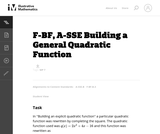Unrestricted Use
CC BY
Rating

This task is for instructional purposes only and builds on ``Building an explicit quadratic function.''

نوع المادة:
Activity/Lab
Provider:
Illustrative Mathematics
Provider Set:
Illustrative Mathematics
المؤلف:
Illustrative Mathematics
08/20/2012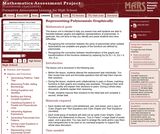Only Sharing Permitted
CC BY-NC-ND
Rating

This lesson unit is intended to help teachers assess how well students are able to translate between graphs and algebraic representations of polynomials. In particular, this unit aims to help you identify and assist students who have difficulties in: recognizing the connection between the zeros of polynomials when suitable factorizations are available, and graphs of the functions defined by polynomials; and recognizing the connection between transformations of the graphs and transformations of the functions obtained by replacing f(x) by f(x + k), f(x) + k, -f(x), f(-x).

Material Type:
Assessment
Lesson Plan
Provider:
Shell Center for Mathematical Education
U.C. Berkeley
Provider Set:
Mathematics Assessment Project (MAP)
04/26/2013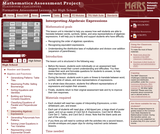Only Sharing Permitted
CC BY-NC-ND
Rating

This lesson unit is intended to help teachers assess how well students are able to translate between words, symbols, tables, and area representations of algebraic expressions. It will help teachers to identify and support students who have difficulty in: recognizing the order of algebraic operations; recognizing equivalent expressions; and understanding the distributive laws of multiplication and division over addition (expansion of parentheses).

Material Type:
Assessment
Lesson Plan
Provider:
Shell Center for Mathematical Education
U.C. Berkeley
Provider Set:
Mathematics Assessment Project (MAP)
04/26/2013Only Sharing Permitted
CC BY-NC-ND
Rating

This lesson unit is intended to help teachers assess how well students are able to interpret exponential and linear functions and in particular to identify and help students who have the following difficulties: translating between descriptive, algebraic and tabular data, and graphical representation of the functions; recognizing how, and why, a quantity changes per unit intervale; and to achieve these goals students work on simple and compound interest problems.

نوع المادة:
التقييم
Lesson Plan
Provider:
Shell Center for Mathematical Education
U.C. Berkeley
Provider Set:
Mathematics Assessment Project (MAP)
04/26/2013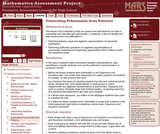Only Sharing Permitted
CC BY-NC-ND
Rating

This lesson unit is intended to help you assess how well students are able to manipulate and calculate with polynomials. In particular, it aims to identify and help students who have difficulties in: switching between visual and algebraic representations of polynomial expressions; and performing arithmetic operations on algebraic representations of polynomials, factorizing and expanding appropriately when it helps to make the operations easier.

Material Type:
Assessment
Lesson Plan
Provider:
Shell Center for Mathematical Education
U.C. Berkeley
Provider Set:
Mathematics Assessment Project (MAP)
04/26/2013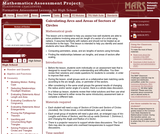Only Sharing Permitted
CC BY-NC-ND
Rating

This lesson unit is intended to help teachers assess how well students are able to solve problems involving area and arc length of a sector of a circle using radians. It assumes familiarity with radians and should not be treated as an introduction to the topic. This lesson is intended to help teachers identify and assist students who have difficulties in: Computing perimeters, areas, and arc lengths of sectors using formulas and finding the relationships between arc lengths, and areas of sectors after scaling.

Material Type:
Assessment
Lesson Plan
Provider:
Shell Center for Mathematical Education
U.C. Berkeley
Provider Set:
Mathematics Assessment Project (MAP)
04/26/2013Only Sharing Permitted
CC BY-NC-ND
Rating

This lesson unit is intended to help teachers assess how well students are able to understand what the different algebraic forms of a quadratic function reveal about the properties of its graphical representation. In particular, the lesson will help teachers identify and help students who have the following difficulties: understanding how the factored form of the function can identify a graphŐs roots; understanding how the completed square form of the function can identify a graphŐs maximum or minimum point; and understanding how the standard form of the function can identify a graphŐs intercept.

Material Type:
Assessment
Lesson Plan
Provider:
Shell Center for Mathematical Education
U.C. Berkeley
Provider Set:
Mathematics Assessment Project (MAP)
04/26/2013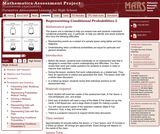Only Sharing Permitted
CC BY-NC-ND
Rating

This lesson unit is intended to help teachers assess how well students understand conditional probability, and, in particular, to help teachers identify and assist students who have the following difficulties: representing events as a subset of a sample space using tables and tree diagrams; and understanding when conditional probabilities are equal for particular and general situations.

Material Type:
Assessment
Lesson Plan
Provider:
Shell Center for Mathematical Education
U.C. Berkeley
Provider Set:
Mathematics Assessment Project (MAP)
04/26/2013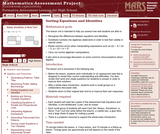Only Sharing Permitted
CC BY-NC-ND
Rating

This lesson unit is intended to help teachers assess how well students are able to: recognize the differences between equations and identities; substitute numbers into algebraic statements in order to test their validity in special cases; resist common errors when manipulating expressions such as 2(x Đ 3) = 2x Đ 3; (x + 3)_ = x_ + 3_; and carry out correct algebraic manipulations. It also aims to encourage discussion on some common misconceptions about algebra.

Material Type:
Assessment
Lesson Plan
Provider:
Shell Center for Mathematical Education
U.C. Berkeley
Provider Set:
Mathematics Assessment Project (MAP)